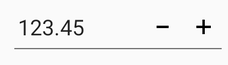# Parsing the Value in Xamarin NumericUpDown (SfNumericUpDown)

19 May 20211 minute to read

The value of NumericUpDown is parsed based on the ParsingMode property. ParsingMode is a type of Parsers; it can be enum of Double and Decimal. You have options to update the value in double or decimal.

The following code demonstrates the decimal parsing mode, which can be set using the ParsingMode property.

``<numeric:SfNumericUpDown x:Name="NumericUpDown" Value="123.45" ParsingMode="Decimal" />``
``````SfNumericUpDown NumericUpDown=new SfNumericUpDown();
NumericUpDown.ParsingMode=Parsers.Decimal;
NumericUpDown.Value = 123.45;
this.Content = NumericUpDown;``````

NOTE

The default value of ParsingMode is Double.